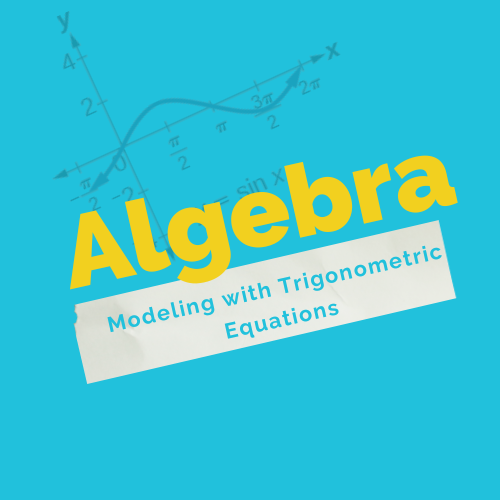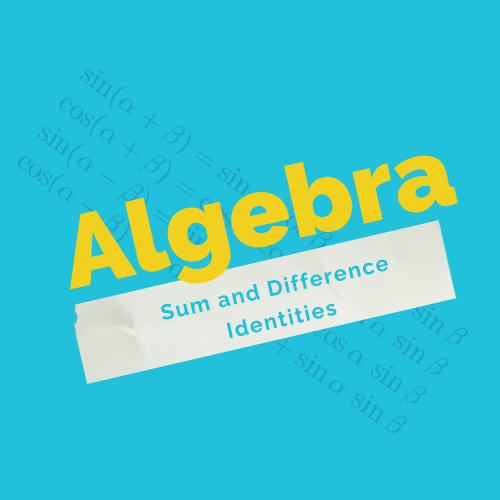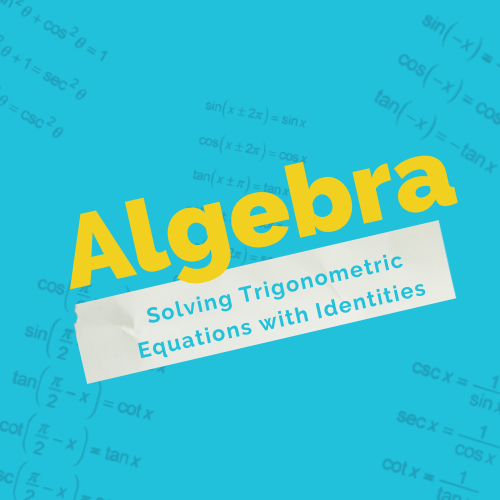Chapter Title ( Trigonometric Identities and Equations )

•Modeling with Trigonometric Equations
Gallery

## Modeling with Trigonometric Equations

In this course you will: Determine the amplitude [...]

Modeling with Trigonometric Equations2022-12-03T18:21:52+00:00
•Solving Trigonometric Equations
Gallery

## Solving Trigonometric Equations

In this course you will: Solve linear trigonometric [...]

Solving Trigonometric Equations2022-12-03T18:24:14+00:00
•Sum-to-Product and Product-to-Sum Formulas
Gallery

## Sum-to-Product and Product-to-Sum Formulas

In this course you will: Express products as [...]

Sum-to-Product and Product-to-Sum Formulas2022-12-03T18:25:28+00:00

## Double-Angle, Half-Angle, and Reduction Formulas

In this course you will: Use double-angle formulas [...]

Double-Angle, Half-Angle, and Reduction Formulas2022-12-03T18:26:23+00:00
•Sum and Difference Identities
Gallery

## Sum and Difference Identities

In this course you will: Use sum and [...]

Sum and Difference Identities2022-12-03T18:27:39+00:00
•Solving Trigonometric Equations with Identities
Gallery

## Solving Trigonometric Equations with Identities

In this course you will: Verify the fundamental [...]

Solving Trigonometric Equations with Identities2022-12-03T18:28:55+00:00
Go to Top